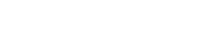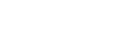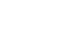# Grounding (Calculations)

(Please see my last art on grounding [methods of refloating])

In the pinnacle or point grounding the basic formula always used is

Change of tide at point of contact = Fall of tide in m =Where, P is the ground reaction on hull by bed after a certain fall of tide. RF is the distance of COF to rock. This same formula can be used to do all the following examples. The ready formulae are however, provided, which can be used by a mariner by programming them appropriately. So, let us look at a few simple but important formulae, which may be used by a mariner to get quick results in case of grounding:

Formula to find up-thrust that will be caused on hull after a certain fall of tide

Ex 1. A fall of tide (FOT) of 80 cm is expected. An isolated rock (pinnacle or centroid of ground reaction) is at a point 40m abaft STEM. Calculate up-thrust after the tide falls. (Pinnacle Grounding)

Soln: RF is distance of rock to COF

Upthrust =Formula to find approximate present up-thrust using free floating drafts and present drafts after fall of tide

Ex 2. Free floating drafts: F 8.00 A9.00 Present draft: F: 6.90 A: 9.20. Find up-thrust (All Groundings).

Soln:

Upthrust =Method to find accurate present up-thrust using free floating drafts and present drafts after fall of tide

Ex 3. Free floating drafts: F: 8.00m A: 9.00m Present draft: F: 6.90m A: 9.20m. Find up-thrust (using tables).

Soln:Hyd draft1 = 8.531m    Hyd draft2 = 8.122m

Δ1 = 19,500t  and Δ2 = 18,440t from hydrostatic tables for initial and final hydrostatic drafts respectively. The up-thrust = 19,500t  – 18,440t = 1060t.

Method to find rise of tide necessary for re-floatation if final drafts after fall of tide is known

Ex 4. If by the method given in question 2 or 3, it is found that the up-thrust is 775t after the fall of tide, with the vessel touching the bed at a point 40 m aft of STEM, the rise of tide necessary for re-floatation can be found as follows: (Pinnacle Grounding).

Soln: RF is distance of rock to COF

Rise of tide in cm =Formula to find the centroid of grounding if fall of tide and final drafts after fall of tide is known

Ex 5. Find the position of centroid of grounding if fall of tide is 80 cm and up-thrust found by the method given in question 2 or 3 is 775t. (Pinnacle Grounding)

Soln: The golden rule is that the change of draft at point of   contact = FOT. If the change of trim is aft ways, the grounding point must be forward of COF and vice versa. The distance of grounding point from COF is given by= 45m

Formula to find amount of ballast to be shifted in order to release the ship from ground.

Ex 6. In the ‘question 1 situation’, how much of ballast must be shifted between the peak tanks located 120m apart to free float the vessel at this stage where the tide is fallen by 80cm. (Pinnacle Grounding).

Soln

Weight to shift =to be shifted from fore peak to aft peak.

Note:

1. 474 x 120 tm is the trimming moment required.

2. There is no change of tide, hence change of draft at R is purely due change due trim alone. I.e. trr = 0.80 butAlso, trc =(See the methods used by salvage experts in my next article)

(You may also visit my youtube videos @captsschaudhari.com)